# Heap Sort

Heap sort is a sorting algorithm that sorts data in ascending or descending order using the input data. It is a tree-like structure created from the input data. It’s similar to selection sorting, in which we find the smallest element first and place it at the top. The process is repeated for the remaining elements.To complete the sorting, the algorithm requires 3 steps:

1. With input data, create a heap
2. The heap’s elements are sorted in ascending order.
3. Swap the root node with the last node in the heap and delete the last node.

Before proceeding further, let’s understand Complete Binary Tree and Heapify.

### Complete Binary Tree

It is a tree in which all levels are completely filled. It has one exception in the lowest level, which is possibly filled from the left. The leaf node must all lean to the left. A complete binary tree does not have to be a full binary tree because the last leaf element may not have the right sibling.

This is a Full Binary Tree but not Complete Binary Tree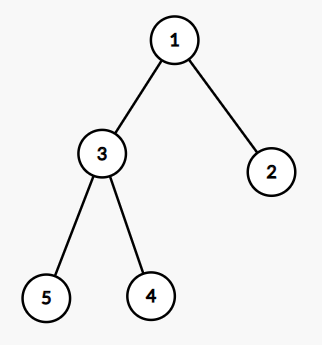Complete Binary Tree but not a Full Binary Tree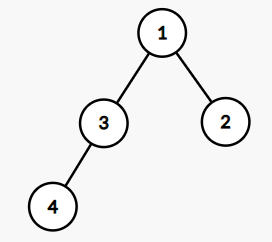This is a Full Binary Tree and a Complete Binary Tree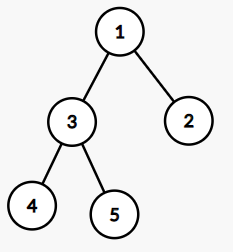### Heapify:

Heapify is the process of converting a binary tree into a heap data structure. A Min-Heap or a Max-Heap can be made with it. Let’s try to create a max-heap from a given array of elements.

```Suppose arr=[1, 3, 5, 4, 6, 13, 10, 9, 8, 15, 17] .
Root of the tree is at 0th Index.
Left child of ith node = 2*i+1
Right child of ith node = 2*i+2
Parent of ith node = (i-1)/2
```

Corresponding Binary Tree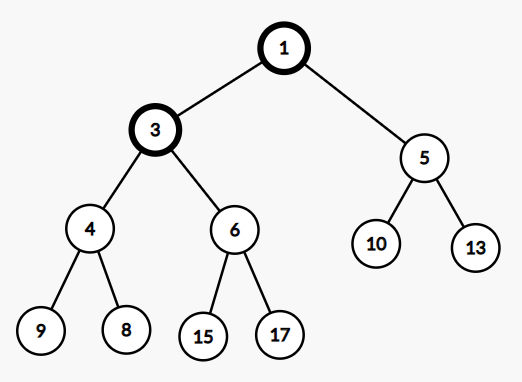Last non-leaf node = (11/2)-1 = 4 . Therefore, last non-leaf node = 6 (arr)  . So we will start to heapify only the [1,3,5,4,6] parts. We will go level-wise from bottom to up and check whether the current node’s left or right children are greater or not. If anyone of them is greater, we will swap

17 is the largest among 6,15. So will heapify 6 and 17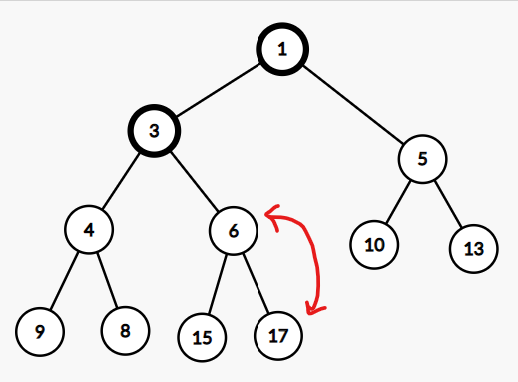9 is the largest among 8,4. So will heapify 9 and 4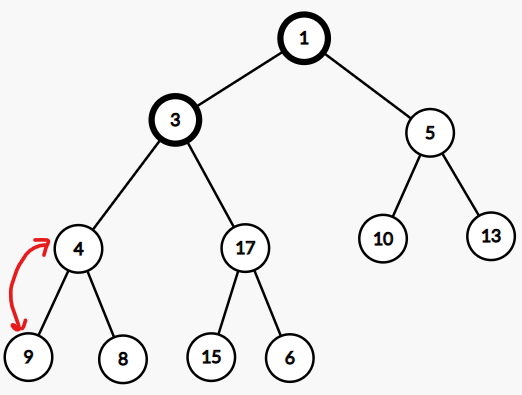13 is the largest among 5,10. So will heapify 5 and 13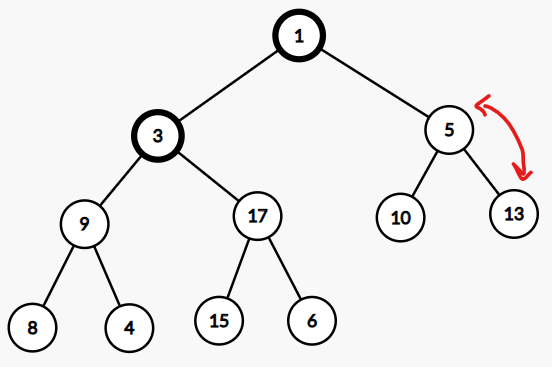17 is the largest, so will swap 3 and 17 but 15 > 3 . So will swap 15 and 3 after swapping 3 and 17.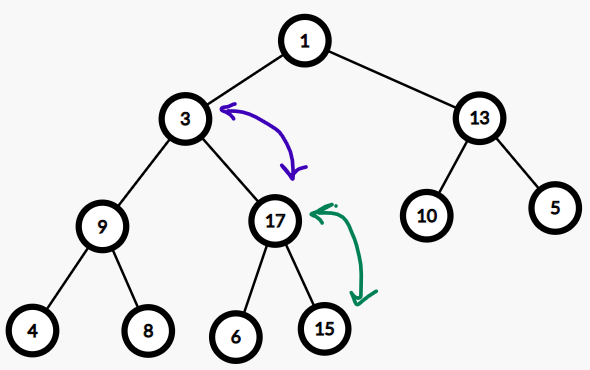1 is swapped with 17, then 1 is swapped with 15. Then finally 1 and 6 are swapped.Finally, Max-Heap will look like the below: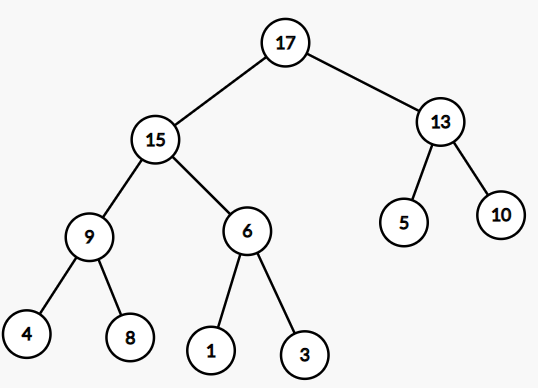Code:

## C++ Code

``````#include <bits/stdc++.h>
using namespace std ;

void Heapify(vector<int>&arr, int n , int index) {

int right = 2 * index + 2;
int left = 2 * index + 1;
int largestNode = index  ;

if (left < n  && arr[left] > arr[largestNode]) {
largestNode = left ;
}

if (right < n && arr[right] > arr[largestNode]) {
largestNode = right  ;
}

if (largestNode != index) {
swap(arr[index], arr[largestNode])  ;
Heapify(arr, n, largestNode)  ;
}
}
void createHeap(vector<int>&arr, int n) {

int start = (n / 2) - 1 ;

for (int index = start ; index >= 0; index--) {
Heapify(arr, n, index)  ;
}
}
void printHeap(vector<int>&arr, int n) {

for (int i = 0; i < n; i++) {
cout << arr[i] << " "  ;
}
cout << "\n"  ;
}
int main() {

vector<int>arr = {1, 3, 5, 4, 6, 13, 10, 9, 8, 15, 17} ;

int n = arr.size()   ;

createHeap(arr, n)  ;
cout << "Heap in form of Array:-\n"  ;
printHeap(arr, n)  ;
}
``````

Output:

Heap in form of Array:-
17 15 13 9 6 5 10 4 8 3 1

## Java Code

``````import java.util.*;

class TUF{
static void Heapify(int[] arr, int n , int index) {

int right = 2 * index + 2;
int left = 2 * index + 1;
int largestNode = index  ;

if (left < n  && arr[left] > arr[largestNode]) {
largestNode = left ;
}

if (right < n && arr[right] > arr[largestNode]) {
largestNode = right  ;
}
int temp;
if (largestNode != index) {
temp=arr[index];
arr[index]=arr[largestNode];
arr[largestNode]=temp;
Heapify(arr, n, largestNode)  ;
}
}
static void createHeap(int[] arr, int n) {

int start = (n / 2) - 1 ;

for (int index = start ; index >= 0; index--) {
Heapify(arr, n, index)  ;
}
}
static void printHeap(int[] arr, int n) {

for (int i = 0; i < n; i++) {
System.out.print(arr[i]+" ")  ;
}
System.out.println();
}
public static void main(String args[]) {

int arr[] = {1, 3, 5, 4, 6, 13, 10, 9, 8, 15, 17} ;

int n = arr.length   ;

createHeap(arr, n)  ;
System.out.println("Heap in form of Array:-")  ;
printHeap(arr, n)  ;
}
}``````

Output:

Heap in form of Array:-
17 15 13 9 6 5 10 4 8 3 1

Working of Heap Sort :

1. When it comes to the maximum heap, the root node stores the highest element.
2. Swap: We need to remove the root element and replace it with the tree’s last element (heap).
3. Remove: Reduce the heap’s size by one.
4. Heapify: Heapify the root element once more, this time to the highest element.

The procedure is repeated until the entire list has been sorted.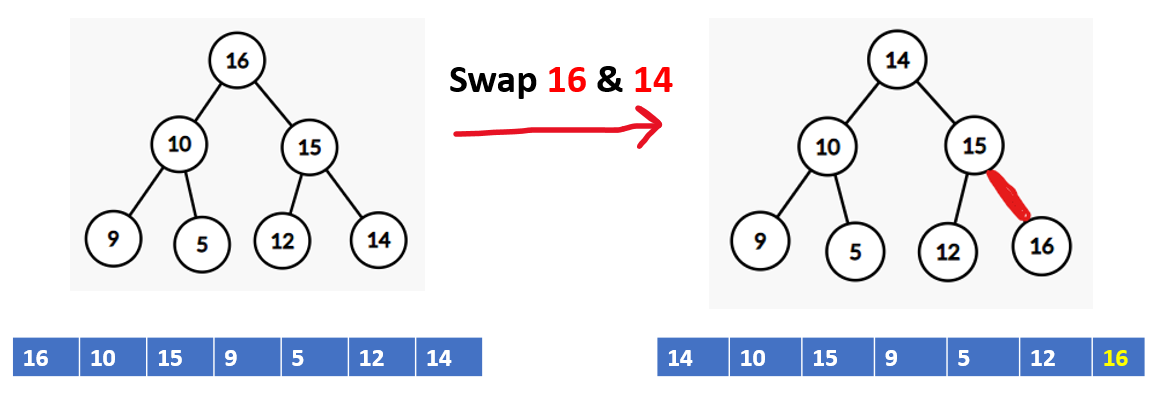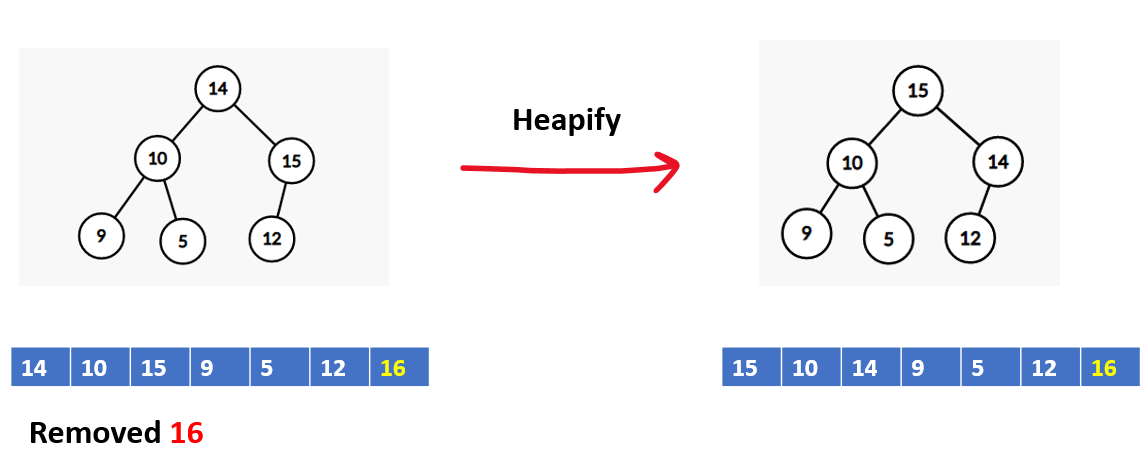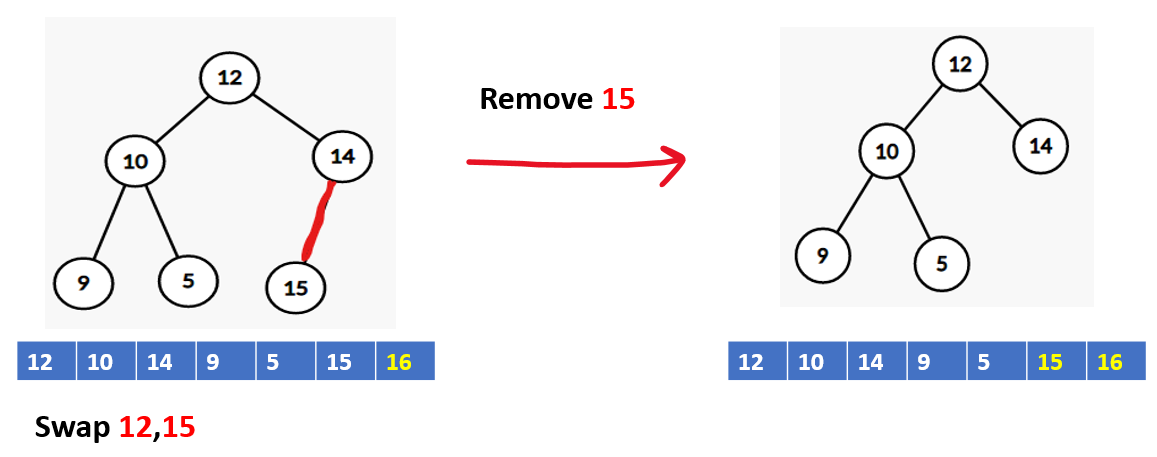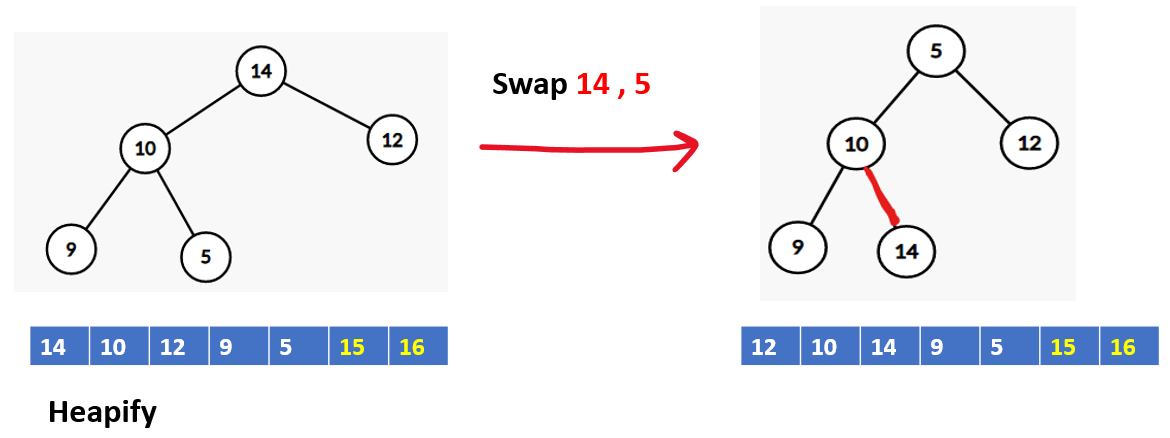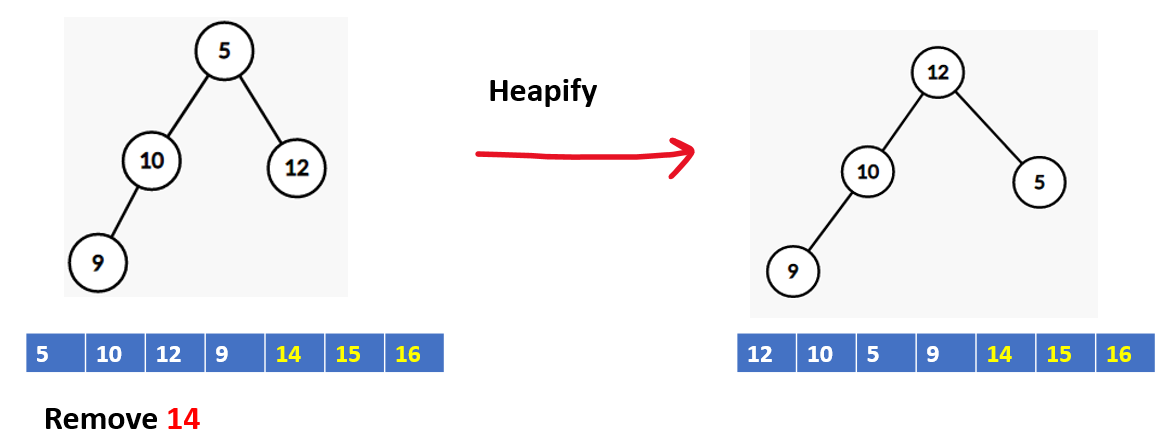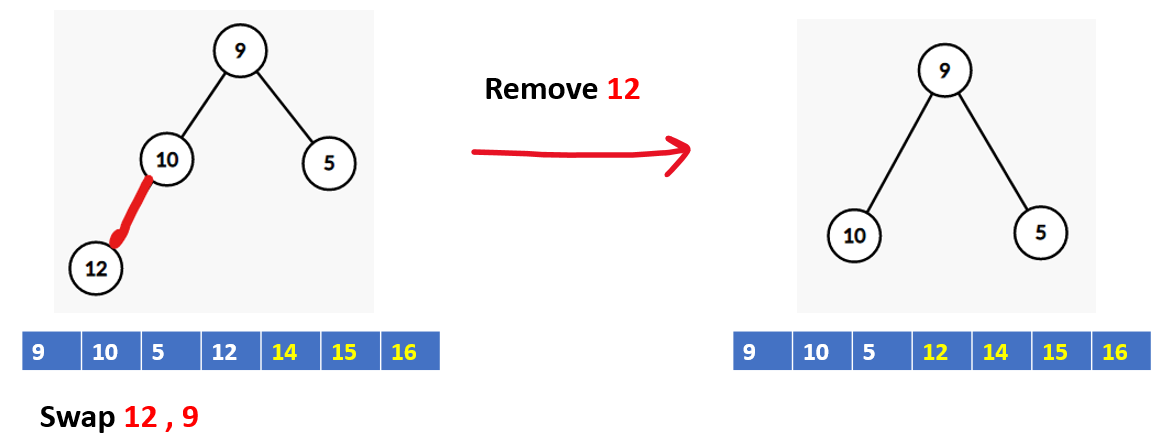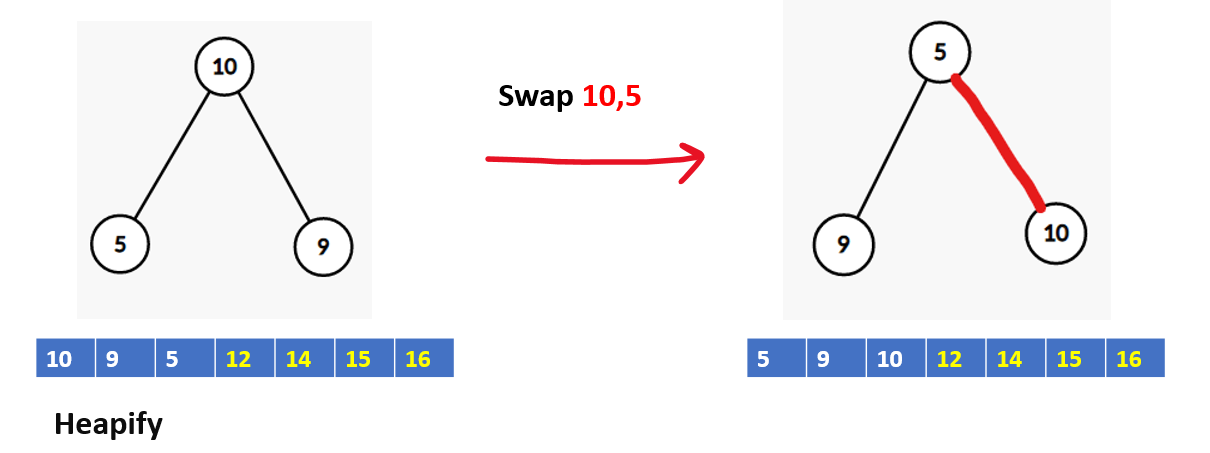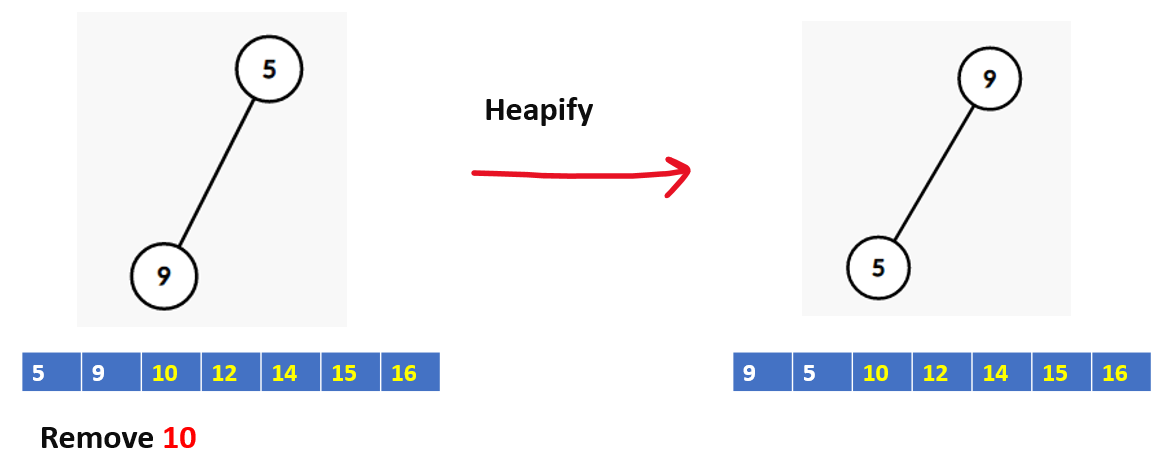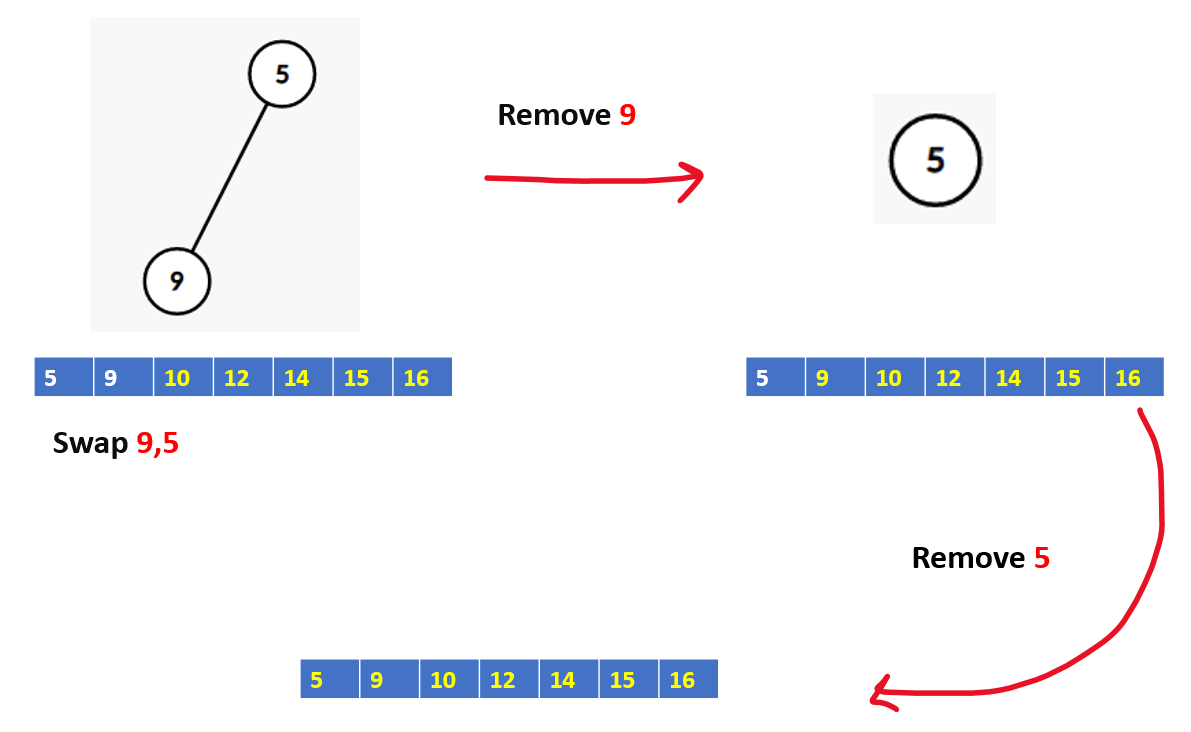Code:

## C++ Code

``````#include <bits/stdc++.h>
using namespace std ;

void Heapify(vector<int>&arr, int n , int index) {

int right = 2 * index + 2;
int left = 2 * index + 1;
int largestNode = index  ;

if (left < n  && arr[left] > arr[largestNode]) {
largestNode = left ;
}

if (right < n && arr[right] > arr[largestNode]) {
largestNode = right  ;
}

if (largestNode != index) {
swap(arr[index], arr[largestNode])  ;
Heapify(arr, n, largestNode)  ;
}
}
void heapSort(vector<int>&arr, int n) {

for(int i=(n/2)-1 ; i>=0; i--){
Heapify(arr,n,i)  ;
}
for(int i=n-1;i>0;i--){
swap(arr,arr[i])  ;
Heapify(arr,i,0)  ;
}
}
void printHeap(vector<int>&arr, int n) {

for (int i = 0; i < n; i++) {
cout << arr[i] << " "  ;
}
cout << "\n"  ;
}
int main() {

vector<int>arr = {1, 3, 5, 4, 6, 13, 10, 9, 8, 15, 17} ;

int n = arr.size()   ;

heapSort(arr, n)  ;
cout << "Sorted Array:-\n"  ;
printHeap(arr, n)  ;
}
``````

Output:

Sorted Array:-
1 3 4 5 6 8 9 10 13 15 17

Time complexity: O(nlogn), Heapify – O(logn), and Creating heap takes – O(n)

Space complexity: O(1), We stored the item we removed in the space that was left over at the end of the input array.

## Java Code

``````import java.util.*;

class TUF{
static void Heapify(int[] arr, int n , int index) {

int right = 2 * index + 2;
int left = 2 * index + 1;
int largestNode = index  ;

if (left < n  && arr[left] > arr[largestNode]) {
largestNode = left ;
}

if (right < n && arr[right] > arr[largestNode]) {
largestNode = right  ;
}

if (largestNode != index) {
int temp=arr[index];
arr[index]=arr[largestNode];
arr[largestNode]=temp;
Heapify(arr, n, largestNode)  ;
}
}
static void heapSort(int[] arr, int n) {

for(int i=(n/2)-1 ; i>=0; i--){
Heapify(arr,n,i)  ;
}
for(int i=n-1;i>0;i--){
int temp=arr;
arr=arr[i];
arr[i]=temp;
Heapify(arr,i,0)  ;
}
}
static void printHeap(int[] arr, int n) {

for (int i = 0; i < n; i++) {
System.out.print(arr[i]+" ")  ;
}
System.out.println() ;
}
public static void main(String args[]) {

int arr[] = {1, 3, 5, 4, 6, 13, 10, 9, 8, 15, 17} ;

int n = arr.length   ;

heapSort(arr, n)  ;
System.out.println("Sorted Array:-")  ;
printHeap(arr, n)  ;
}
}``````

Output:

Sorted Array:-
1 3 4 5 6 8 9 10 13 15 17

Time complexity: O(nlogn), Heapify – O(logn), and Creating heap takes – O(n)

Space complexity: O(1), We stored the item we removed in the space that was left over at the end of the input array.

Special thanks to Shreyas Vishwakarma for contributing to this article on takeUforward. If you also wish to share your knowledge with the takeUforward fam, please check out this article